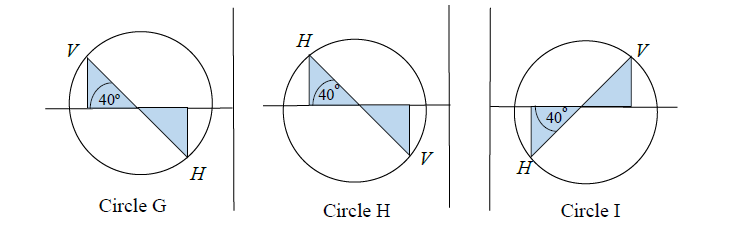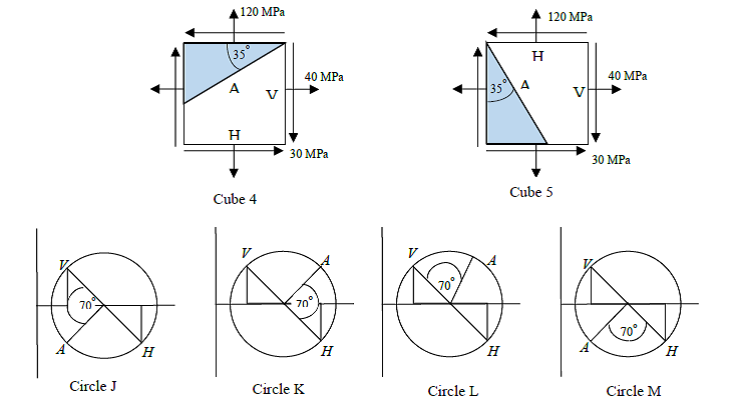# 8.2 Mohr Circle for Stress

NameEmail
1) Cube 1  is associated with the
2) Cube 2  is associated with the
3) Cube 3  is associated with the
4)V and H refer to the vertical and horizontal plane, respectively in circle G. The principal angle 1 is
5) V and H refer to the vertical and horizontal plane, respectively in circle H. The principal angle 1 is
6) V and H refer to the vertical and horizontal plane, respectively in circle I. The principal angle 1 is
7)Cube 4 is associated with Mohr’s
8) Cube 5 is associated with Mohr’s
9) The coordinates of the center of the Mohr’s circle corresponding to cube 4 (or cube 5) is
10) The radius of the Mohr’s circle corresponding to cube 4 (or cube 5) is